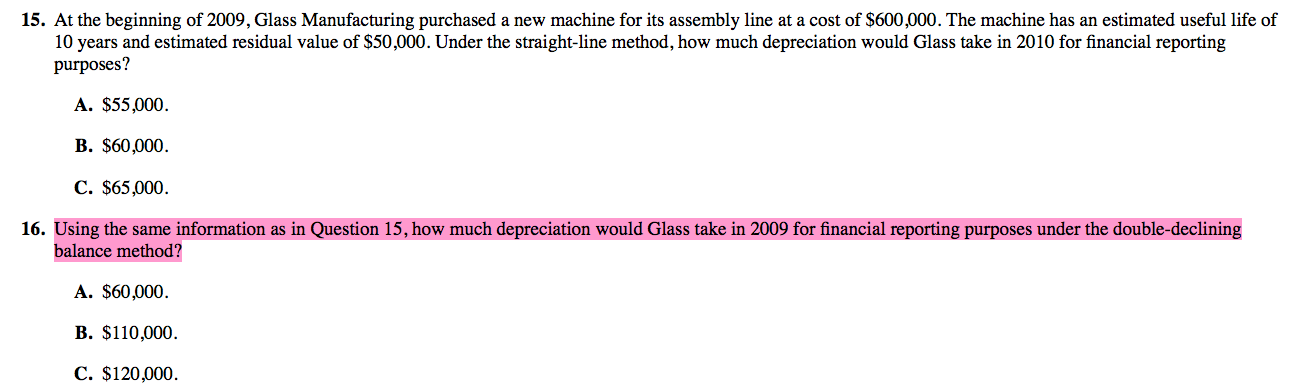CFA CFA Level 1 Double declining depreciation vs depreciation expense

# Double declining depreciation vs depreciation expense

• Author
Posts
• 1Hello everyone, thank you in advance to those who attempt to answer my question, truly appreciate the time and effort you are taking to help me 🙂

I just have a quick question. The question above is from CFAI end of the chapter. For question number 16, why is it that the double declining depreciation formula does not deduct the estimated residual value? I used the formula in our textbook and got B but the correct answer is C where the formula is just 600,000 x 2. Can someone explain why?

Thank you!

• 5

You are brilliant! thank you so much! 🙂

• 3

Not sure If I can help, but the way I would approach the problem is.

Residual value (Whats left) – The amount you will value your Asset at the end of the term.

Therefore Straight line –  You would like your asset to be valued at 50K after 10 years. Therefore 600K-50K = (550000/10) This will be your depreciation for ten years which will result in 50K left over(Salvage value) It’s a constant 50K every year. Therefore Net Income will be the same for all years if all other variable stay the same.

With double declining you want to accelerate your depreciation.
Therefore you would take the period they want to depreciate over (10yr) and you would like to depreciate it twice as fast (Double Declining).
So, (10/2 = 0.2)

Take your value 600K *0.2 = 120,000 for first year
2009 Yr1 = 120,000
2010 Yr2 = (600-120)*0.2 = 96000   (Cost – Accumulated Depreciation)
2011 Yr3 = (600-120-96)*0.2 = 76800
2012 Yr4 = (600-120-96-76.8)*0.2 = 61440
2013 Yr5 = (600-120-96-76.8-61.4)*0.2 = 49160

and so on…

REMEMBER!! Once you hit 50K on your Asset value, you cannot not depreciate further than that.!

Net Income would increase as the years go by, if everything stays constant

Accumulated depreciation is the total amount for all depreciated values
Residual Value is the amount you want your asset to be valued at the end of the term.

Hope this helps

• 3

Simple as that DDB=2/useful life (Cost- Acc Dep )

in 2009 we don’t have any acc dep as it’s the beg year so the answer will be:

2/10 (600,000) =120,000

Hope that help.

• 2

for accelerating methods u count liek this:

600k / 10periods  *2 (double declining – 120k

• 2

in accelerating methods u dont substract residual value

• 1